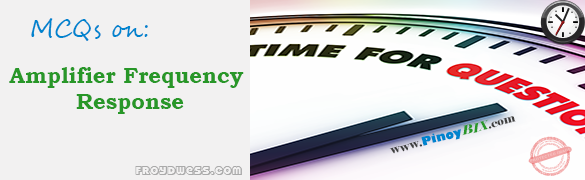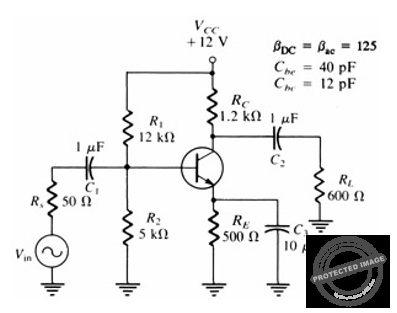# MCQs in Amplifier Frequency Response

(Last Updated On: December 8, 2017)This is the Multiple Choice Questions in Amplifier Frequency Response from the book Electronic Devices – Electron Flow Version and Conventional Current Version 8th Edition by Thomas L. Floyd. If you are looking for a reviewer in Electronics Engineering this will definitely help. I can assure you that this will be a great help in reviewing the book in preparation for your Board Exam. Make sure to familiarize each and every questions to increase the chance of passing the ECE Board Exam.

### Practice Exam Test Questions

Choose the letter of the best answer in each questions.

1. The lower critical frequency is also known as the

• A. break frequency.
• B. cutoff frequency.
• C. corner frequency.
• D. all of the above

2. What term means that the midrange voltage gain is assigned a value of 1 (or 0 dB)?

• A. critical
• B. Miller
• C. normalized
• D. corner

3. In a multistage amplifier, the overall frequency response is determined by the

• A. frequency response of each stage depending on the relationships of the critical frequencies.
• B. frequency response of the first amplifier.
• C. frequency response of the last amplifier.
• D. lower critical frequency of the first amplifier and the upper critical frequency of the final amplifier.

4. The frequency response of an amplifier can be determined using the step response method, and measuring the output rise/fall times between

• A. 0% and 100% response.
• B. 10% and 90% response.
• C. 25% and 75% response.
• D. five time constant responses.

5. For low-frequency response, all RC circuits in an amplifier may not have the same critical frequency. Which RC response will determine the critical frequency of the amplifier?

• A. the lowest frequency
• B. the center frequency
• C. the highest frequency
• D. the bypass frequency

6. An amplifier has an output voltage of 7.6 V p-p at the midpoint of the frequency range. What is the output at fc?

• A. 3.8 V p-p
• B. 3.8 Vrms
• C. 5.4 Vrms
• D. 5.4 V p-p

7. An amplifier has an input signal voltage of 0.054 mV. The output voltage is 12.5 V. The voltage gain in dB is

• A. 53.6 dB.
• B. 107.3 dB.
• C. 231 dB.
• D. 116 dB.

8. A certain amplifier has a bandwidth of 22.5 kHz with a lower cutoff frequency of 600 Hz. What is the value of fcu?

• A. 600 Hz
• B. 22.5 kHz
• C. 23.1 kHz
• D. 21.9 kHz

9. An amplifier has a Rin = 1.2 kΩ. The coupling capacitor is 1 µF. Determine the approximate lower cutoff frequency.

• A. 133 Hz
• B. 1.33 kHz
• C. 13.3 kHz
• D. 133 kHz

10. An RC network has values of R = 1.2 kΩ and C = 0.22 µF. Find fc.

• A. 3.79 kHz
• B. 1.89 kHz
• C. 603 Hz
• D. 60 Hz

11. Refer to Figure 10-1. The capacitor C1 affectsFigure 10-1

• A. high-frequency response.
• B. low-frequency response.
• C. midrange response.
• D. nothing.

12. Refer to Figure 10-1. The capacitor C3 affects

• A. high-frequency response.
• B. low-frequency response.
• C. midrange response.
• D. nothing.

13. Refer to Figure 10-1. The capacitor Cbe affects

• A. high-frequency response.
• B. low-frequency response.
• C. midrange response.
• D. nothing.

14. Refer to Figure 10-1. If RL decreases in value, the output voltage will

• A. increase.
• B. decrease.
• C. remain the same.

15. Refer to Figure 10-1. The upper cutoff frequency of this amplifier is 22 kHz. The output at that frequency is 6.71 V p-p. What is the output voltage at 220 kHz?

• A. 9.49 V p-p
• B. 6.71 V p-p
• C. 0.671 V p-p
• D. 0.0671 V p-p

16. Refer to Figure 10-1. You measure an output voltage at the lower cutoff frequency of 3.42 V p-p. The output voltage at the upper cutoff frequency will be

• A. 2.42 V p-p.
• B. 3.42 V p-p.
• C. 6.84 V p-p.
• D. 6.84 Vrms.

17. Refer to Figure 10-1. The output voltage at fcl = 12 mV. What is the output voltage at the midpoint frequency?

• A. 12 mV
• B. 12 mV p-p
• C. 16.97 mV
• D. 8.48 mV

18. Refer to Figure 10-1. You are attempting to determine the lower cutoff frequency of this amplifier in the lab. As you change the input frequency and measure the output signal, you must remember to

• A. set the oscilloscope to DC.
• B. maintain the input voltage constant.
• C. keep a constant temperature.
• D. watch for a change of β.

19. An RC network has a roll-off of 20 dB per decade. What is the total attenuation between the output voltage in the midrange of the passband as compared to the output voltage at a frequency of 10 times fc?

• A. –3 dB
• B. –20 dB
• C. –23 dB
• D. –43 dB

20. A roll-off of 20 dB per decade is equivalent to a roll-off of _____ per octave.

• A. 3 dB
• B. 13 dB
• C. 12 dB
• D. 6 dB

21. Internal transistor junction capacitances affect the high-frequency response of amplifiers by

• A. reducing the amplifier’s gain.
• B. introducing phase shift as the signal frequency increases.
• C. having no effect.
• D. reducing the amplifier’s gain and introducing phase shift as the signal frequency increases.

22. ______ frequencies are values of frequency at which the RC circuits reduce the voltage gain to 70.7% of its midrange value.

• A. Critical
• B. Cutoff
• C. Corner
• D. all of the above

23. It is often convenient in amplifiers to assign a certain value of gain as the _____ dB reference.

• A. 0
• B. 1
• C. 6
• D. 10
• E. 20

24. Doubling the voltage gain causes a _____ dB ______.

• A. 10, increase
• B. 6, increase
• C. 10, decrease
• D. 6, decrease

25. Halving the power corresponds to a _____ dB ______.

• A. 3, decrease
• B. 10, decrease
• C. 3, increase
• D. 10, increase

26. Each RC circuit causes the gain to drop at a rate of _____ dB/decade.

• A. 20
• B. 10
• C. 6
• D. none of the above

27. A ten-times change in frequency is called a(n)

• A. octave.
• C. decimal.
• D. none of the above

28. A two-times change in frequency is called a(n)

• A. binave.
• B. octave.
• D. none of the above

29. The phase shift through the input of an RC circuit approaches _____° as the frequency approaches zero.

• A. 0
• B. 45
• C. 180
• D. 90

30. What is the method that can be used to determine the values of fcl and fcu of an amplifier?

• A. five time constants
• B. step-response
• C. sinusoidal

TRUE/FALSE

1. Coupling and bypass capacitors affect the high-frequency response of an amplifier.

• A. True
• B. False

2. Internal transistor capacitances affect the high-frequency response of amplifiers.

• A. True
• B. False

3. At the cutoff frequency the output is down 20 dB.

• A. True
• B. False

4. A decade of frequency change is a change by a factor of 10.

• A. True
• B. False

5. Bandwidth is the difference between fcl and fcu.

• A. True
• B. False

6. The basis for the decibel unit stems from the logarithmic response of the human ear to the intensity of sound.

• A. True
• B. False

7. The term "dBm" means decibels referenced to 1 mW of power.

• A. True
• B. False

8. One characteristic of amplifiers is that the sum of the voltage gain and the bandwidth is always constant when the roll-off is –20 dB/decade.

• A. True
• B. False

9. The lower and upper critical frequencies of an amplifier can be determined using the step-response method.

• A. True
• B. False

10. Miller’s theorem is used in the high-frequency analysis of BJTs and FETs.

• A. True
• B. False

Rate this: